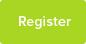# Decompose ((8x + 1)/((x+2)*(x-1))) Into Partial Fractions

Here is an exemplar solution for the above question.

Date : 25/04/2019### Author Information

Uploaded by : Priyash
Uploaded on : 25/04/2019
Subject : Maths

So here we have a question concerning partial fractions. This is a common and slightly tricky question in which we have to decompose a complex fraction into partial fractions.

CONCEPT: The first thing I like to do when confronted with such questions is to ask myself, "How many partial fractions will there be in this case?" And, I get the answer by looking at the highest power of the variable `x` in the denominator. Here I see two factors, namely, (x+2) and (x-1). Now if a polynomial (here the denominator) is made of two factors then the highest power of that polynomial will be? ... Well, 2. Because the highest power is the same as the number of factors. And consequently, we will have two partial fractions.

Now that we have the number of partial fractions, ie. 2, let us write the expression given in the question as a sum of two fractions whose denominators are each of the factors we observed in the previous paragraph and their respective numerators be, say, A and B.

Thus our decomposition looks like:

((8x + 1)/((x+2)*(x-1))) = (A/(x+2)) + (B/(x-1))...................Equation.1

NOTE: I use A and B for two reasons: 1. The largest power of `x` in the numerators of the decomposed partial fractions (or DPFs) is always less than that of the denominators. Here we have the highest power in the DPFs to be 1 respectively. Hence, the largest power of `x` will be: 1 - 1 = 0. Thus the numerators will just be constants. 2. Now I also use two different values for numerators i.e A and B. This is because at this point in our solution there is no guarantee that both the numerators will be the same. Hence for the sake of generality, we must assume them to be different.

Now the only thing left to do is to evaluate the values of A and B and we`d be done!

To do this I add the partial fractions on the Right Hand Side (or RHS) of the `Equation.1` and get a single but complex fraction. The addition is as simple as adding any two generic fractions using the concept of cross multiplication. This shall yield:

RHS = ((A*(x-1)) + (B*(x+2)))/((x+2)*(x-1))

NOTE: that the RHS is equal of the LHS (or Left Hand Side) that being a complex fraction with the numerator (8x+1) and the denominator (x+2)*(x-1). Hence since the RHS and LHS have a common denominator of (x+2)*(x-1). We can cancel it out from the equation and we are left with an equality between (8x+1) and (A*(x-1)) + (B*(x+2))). Thus our new equation is:

(8x + 1)= (A*(x-1)) + (B*(x+2)))..................Equation.2

Now all we have to do is to solve for A and B in this new `Equation.2`

TRICK: I like to do this by putting in values of x and solving the entire equation. Remember, I can do this because there is no restriction on what value of `x` I am allowed to take. Hence I can take any value that I like.

Here comes the trick! Note that if we have x=(1) and x=(-2), then we get two extraordinarily simple equations. i.e:

For x = 1, we have: (8*(1)+1) = (A*(1-1)) + (B*(1+2))) & or, 8+1 = B*(3) or, 9 = 3*B or 3 = B.

Similarly, For x = -2, we have: (8*(-2)+1) = (A*(-2-1)) + (B*(-2+2))) or, -16+1 = A*(-3) or, -15 = A*-3 or A = 5.

Voila, we have the required values of A and B, namely A=5 and B=3.

Finally, this gives us the complete decomposition of the fraction that is:

((8x + 1)/((x+2)*(x-1))) = (5/(x+2)) + (3/(x-1))

:)

This resource was uploaded by: Priyash

### Other articles by this author

© 2005-2019 Tutor Hunt - All Rights Reserved

Privacy Policy | Copyright | Terms of Service
loaded in 0.141 seconds